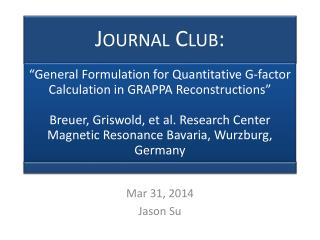DownloadDownload PresentationMar 31, 2014 Jason Su

# Mar 31, 2014 Jason Su

Télécharger la présentation## Mar 31, 2014 Jason Su

- - - - - - - - - - - - - - - - - - - - - - - - - - - E N D - - - - - - - - - - - - - - - - - - - - - - - - - - -
##### Presentation Transcript

1. Mar 31, 2014 Jason Su

2. Motivation • GRAPPA is becoming the dominant form of parallel imaging • Creating reliable g-factor maps is an important tool to have • Allows the evaluation and optimization of different acquisition schemes (CAIPIRINHA or even just how to choose Ry, Rz) • In our studies, we are beginning to wonder what is an acceptable level of acceleration, esp. for visualizing thalamus • G-factor is a critical quantity for this analysis

3. Theory: GRAPPA • Interpolate missing data in k-space from neighboring samples with a kernel • Other coils are considered neighbors • Attain the linear interpolation weights from central ACS region • Here R=3 and using a 2x3(?) kernel • Ssrc [NcNsrc x Nrep] • Strg [NcNtrg x Nrep] • w [NcNtrg x NcNsrc] • Input the source samples from all coils • Output the target points for all coils

4. Theory: GRAPPA • w can be found with pseudoinverse • ACS is our training data • Find the least squares linear regression of the sourceto target points • Predict missing data by sweeping (correlating) over the data • Convolve the flipped kernels, wkl, for all channels • Sum the contributions from all channels to produce one channel of data • Validate against sampled data?

5. Theory: Image Domain Weights • Combine kernels for different target points together into a single kernel by lining up the target points • Get the kernel and image dimensions to match by zero-padding • Then: • By FT and linearity • Here · is element-wise multiplication

6. Theory: Noise Propagation • We are interested in how the noise is modified by the GRAPPA kernel • Replace I, the actual image, with the noise image • The variance of the output noise is then: • By variance of linear combinations

7. Theory: Noise Propagation • I think this would be computed separately for every pixel • Diagonal entry on quadratic form of covariance matrix with some scale factors • Familiar in form to CRLB covariance

8. Theory: G-factor • The g-factor for a coil image • Computed pixel-wise to obtain the whole map

9. Theory: Combined Images • For SOS set pk=Ik*/ISOS • What is ISOS? • SNR-optimal image combination for both nonnormalized and B1-normalized have equivalent g-factor • Requires coil sensitivities

10. Theory: Multiple Kernels • Rm = reduction factor for kernel m • fm= fraction of k-space kernel applied over • gm= g-factor associated with that kernel • Each kernel affects the whole image, so we sum the contributions from each • For ACS data (R=1, g=1, f = ACS/total lines) • What about edge kernels?

11. Methods • Siemens 1.5T • 2D Phantom • TE/TR = 7.1/40ms, α=30deg., bw=100Hz, 256x256 • Noise only image with α=0 to measure noise correlation • R = [2, 3, 4] • 3D In Vivo • MPRAGE: TE/TR = 4.38/1350ms, TR=800ms, α=15deg., bw=180Hz, 256x192x160 • Noise only image with α=0 • Rectangular and CAIPIRINHA sampling, R=2x2 • 3x3x3 kernel with 24x24x32 ACS block • Simulated non-cartesian GRAPPA • Variable density • PROPELLER, R = [2, 3, 4] • Validation against pseudomultiple replica

12. Pseudomultiple Replica • Generate 300+ artificial images by adding bootstrapped noise • Collected noise images are randomly reordered and added to the acquired coil data • Compare analytic g-factor to simulated g-factor

13. Pseudomultiple Replica

14. Results: 2D Phantom • Perfect match • Overestimation without including noise correlation

15. Results

16. Results: In vivo and PROPELLER

17. Discussion • Can be used to identify the optimal reconstruction kernel, acceleration factor, sampling scheme • For multiple kernels: • Can treat kernels that share source points as having uncorrelated noise • Why?